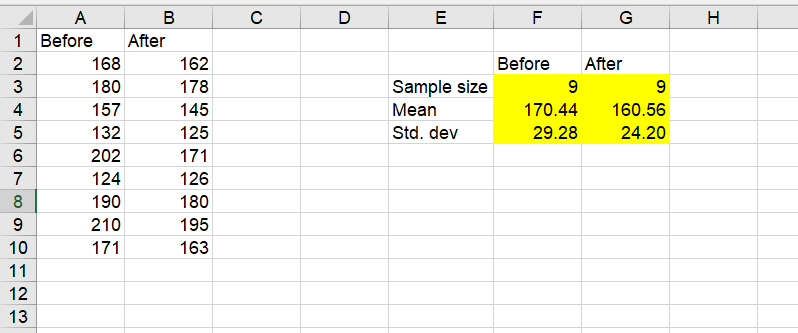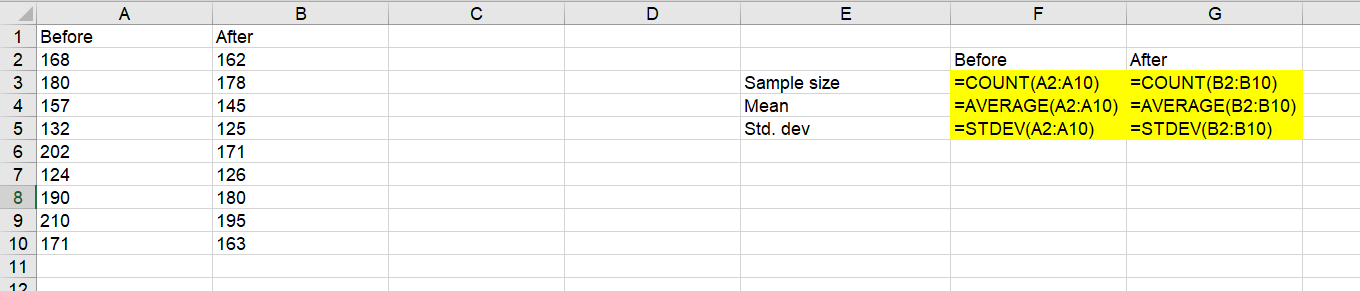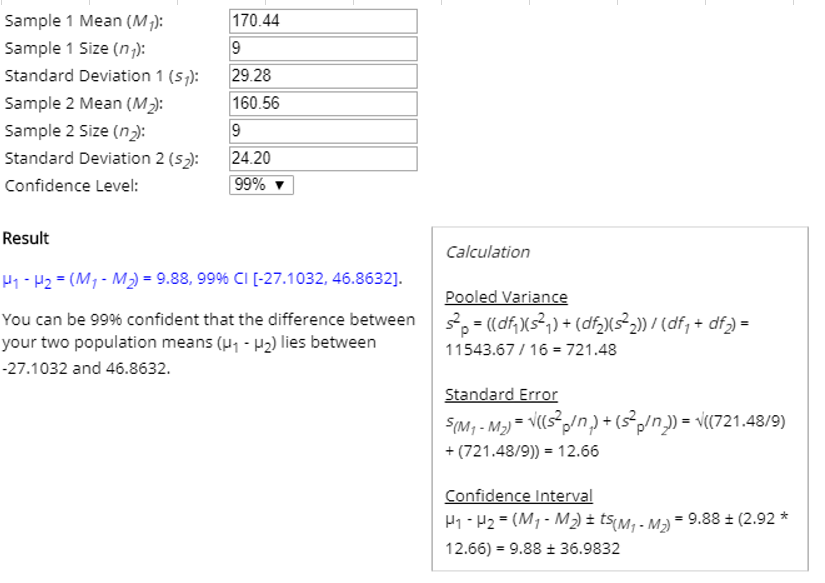##### Question

In: Statistics and Probability

# Construct a confidence interval for ?? the mean of the differences d for the population of...

Construct a confidence interval for ?? the mean of the differences d for the population of paired data. Assume that the population of paired differences is normally distributed. The table shows the weights of 9 subjects before and after following a particular diet for two months. Construct a 99% confidence interval for the mean difference of the “before” minus “after” weights. Round to one decimal place.

 Subject A B C D E F G H I Before 168 180 157 132 202 124 190 210 171 After 162 178 145 125 171 126 180 195 163

## Solutions

##### Expert Solution

Find sample size, mean and standard deviation from both before and after data:Formula Ref:99% Confidence interval mean difference:

a 99% confidence interval for the mean difference of the “before” minus “after” weights are (-27.1, 46.9)## Related Solutions

##### In order to construct a confidence interval for a population mean, it must be the case...
In order to construct a confidence interval for a population mean, it must be the case that the data comes from a population that is normally distributed, or the sample size is large. Group of answer choices True False
##### Assuming that the population is normally​ distributed, construct a 99% confidence interval for the population​ mean,...
Assuming that the population is normally​ distributed, construct a 99% confidence interval for the population​ mean, based on the following sample size of n=7.​ 1, 2,​ 3,4, 5, 6​,and 30   Change the number 30 to 7 and recalculate the confidence interval. Using these​ results, describe the effect of an outlier​ (that is, an extreme​ value) on the confidence interval. Find a 99 % confidence interval for the population mean. ​(Round to two decimal places as​ needed.) Change the number 30...
##### Assuming that the population is normally​ distributed, construct a 9090​% confidence interval for the population mean...
Assuming that the population is normally​ distributed, construct a 9090​% confidence interval for the population mean for each of the samples below. Explain why these two samples produce different confidence intervals even though they have the same mean and range. Sample​ A: 11    22    33    33    66    66    77    88 Full data set Sample​ B: 11    22    33    44    55    66    77    88 Construct a 9090​% confidence interval for the population mean for sample A. nothing less than or equals≤muμless...
##### Assuming that the population is normally​ distributed, construct a 90​% confidence interval for the population mean...
Assuming that the population is normally​ distributed, construct a 90​% confidence interval for the population mean for each of the samples below. Explain why these two samples produce different confidence intervals even though they have the same mean and range. Sample​ A: 1    1    4    4    5    5    8    8    Sample​ B: 1    2    3    4    5    6   7   8 a. Construct a 90​% confidence interval for the population mean for sample A b. Construct a 90​% confidence interval...
##### Assuming that the population is normally​ distributed, construct a 90% confidence interval for the population​ mean,...
Assuming that the population is normally​ distributed, construct a 90% confidence interval for the population​ mean, based on the following sample size of n=7. ​1, 2,​ 3, 4, 5 6, and 24 Change the number 24 to 7 and recalculate the confidence interval. Using these​ results, describe the effect of an outlier​ (that is, an extreme​ value) on the confidence interval.
##### assuming that the population is normally distributed, construct a 99% confidence interval for the population mean,...
assuming that the population is normally distributed, construct a 99% confidence interval for the population mean, based on the following sample size n=6. 1,2,3,4,5 and 29. in the given data, replace the value 29 with 6 and racalculate the confidence interval. using these results, describe the effect of an outlier on the condidence interval, in general find a 99% confidence interval for the population mean, using the formula.
##### Assuming that the population is normally​ distributed, construct a 95​% confidence interval for the population mean...
Assuming that the population is normally​ distributed, construct a 95​% confidence interval for the population mean for each of the samples below. Explain why these two samples produce different confidence intervals even though they have the same mean and range. Sample A   1 1 2 4 5 7 8 8 Sample B   1 2 3 4 5 6 7 8
##### Assuming that the population is normally​ distributed, construct a 99​% confidence interval for the population mean...
Assuming that the population is normally​ distributed, construct a 99​% confidence interval for the population mean for each of the samples below. Explain why these two samples produce different confidence intervals even though they have the same mean and range. Sample​ A: 1   4   4   4   5   5   5   8 Sample B: 1   2   3   4   5   6   7   8 Construct a 99​% confidence interval for the population mean for sample A. less than or equalsmuless than or equals Type...
##### Assuming that the population is normally​ distributed, construct a 95% confidence interval for the population​ mean,...
Assuming that the population is normally​ distributed, construct a 95% confidence interval for the population​ mean, based on the following sample size of n equals 7. ​1, 2,​ 3, 4, 5, 6, 7, and 23 In the given​ data, replace the value 23 with 7 and recalculate the confidence interval. Using these​ results, describe the effect of an outlier​ (that is, an extreme​ value) on the confidence​ interval, in general. Find a 95% confidence interval for the population​ mean, using...
##### Assuming that the population is normally​ distributed, construct a 99% confidence interval for the population mean...
Assuming that the population is normally​ distributed, construct a 99% confidence interval for the population mean for each of the samples below. Explain why these two samples produce different confidence intervals even though they have the same mean and range. SAMPLE A: 1 1 4 4 5 5 8 8 SAMPLE B: 1 2 3 4 5 6 7 8 1.Construct a 99% confidence interval for the population mean for sample A. ( type integers or decimals rounded to two...